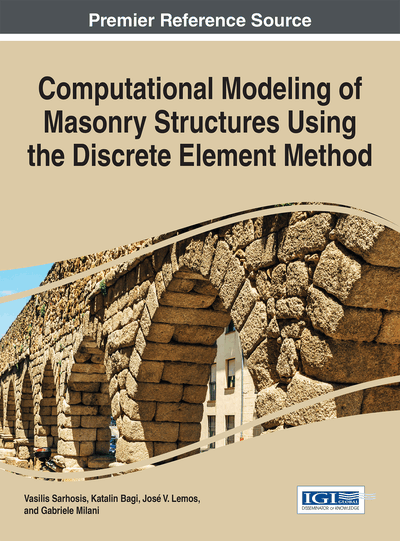# The DDA Method

Katalin Bagi (Budapest University of Technology and Economics, Hungary)
DOI: 10.4018/978-1-5225-0231-9.ch004

## Abstract

“DDA” stands for “Discontinuous Deformation Analysis”, suggesting that the displacement field of the analyzed domain shows abrupt changes on the element boundaries in the model. This chapter introduces the theoretical fundaments of DDA: mechanical characteristics of the elements together with the basic degrees of freedom, contact behavior, the equations of motion and their numerical integration with the help of Newmark's beta-method taking into account contact creation, loss and sliding with the help of an open-close iteration technique. Finally, a short overview on practical and scientific applications for masonry structures is given.
Chapter Preview
Top

## The Elements

The elements in Shi’s model have arbitrary polyhedral shapes. Each element has a reference point, which is the centre of gravity of the element. Denote the position of the reference point of element p by (xp, yp, zp).

The element can translate and rotate as a rigid body (6 degrees of freedom); and in addition to that, a uniform deformation field (characterized by the usual small strain tensor) belongs to the element (another 6 degrees of freedom). The element has altogether 12 degrees of freedom, and the 12 unknown kinematic characteristics are summarized into the generalized displacement vector of the element:

Assuming small displacements, the translations of an arbitrary (x, y, z) point on the element can uniquely be determined with the help of the up generalized displacement vector of the element:

or shortly:

.

Note that these relations are valid for small incremental rotations only (the issue of large rotations will be discussed at the end of Section 2).

This translation vector is particularly important for those points on the element boundary where a contact is formed with a neighbouring element. The difference between the translations of the two material points belonging to the two elements that form the contact is considered the contact deformation. This relative translation determines the forces transmitted through the contact, according to the contact constitutive relations.

Note that the role of matrix T is similar to that of the transition matrix in FEM; the difference is that in DDA the deformation of the element is also taken into account.

## Complete Chapter List

Search this Book:
Reset